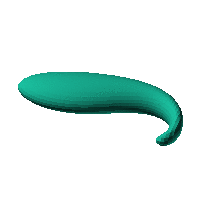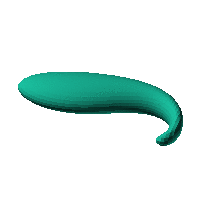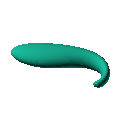Blob Farm# blob57The blob in all its glory:This is a simple ellipsoid swirled at the end. I wonder how you could make it into a case.

 Click on the snapshot to download the blob's stl file.Octave Code:
```  # name of the blob
project = "blob39";

# function at origin must be <0, and >0 far enough away.  w=0 defines the surface
function w = f(x2,y2,z2,c,r)
x = (x2-c(1))/r(1);
y=(y2-c(2))/r(2);
z=(z2-c(3))/r(3);

px1 = 8.0;  py1 =0; # position of swirl
t1 = 2.5;  # effective width of swirl
angle1 = 4; # angle of swirl at the center
one_mat = 0*x+1; # matrix of the right size, but all elements are set to 1.0
an = angle1*(one_mat./(1.0+((x-px1)/t1).^2+((y-py1)/t1).^2));
x3=px1+(x-px1).*cos(an)-(y-py1).*sin(an);
y3=py1+(y-py1).*cos(an)+(x-px1).*sin(an);
z3=z;

w=((x3/3).^2+(y3).^2+(z3.*(1.0+0.0*x3)).^2)-6; # ellipsoid

endfunction;

c_outer = [0,0,0];
r_outer = [14,18,10];

xmin = -150;  # avoid the origin because the inverse of the gradient is NaN there
xmax = 150;

step = 4;  # grid pitch in mm  start with 4mm to see the shape quickly.  Once you have it just right, change to 2mm for printing

source("../octave/func2stl_v01.m");  # do all the calculations
```
GNU Octave